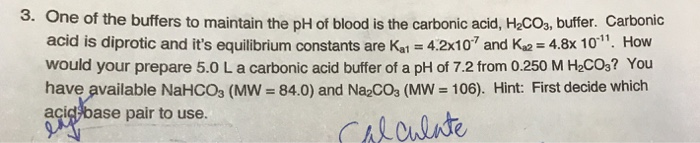# Question & Answer: One of the buffers to maintain the pH of blood is the carbonic acid, H_2CO_3, buffer. Carbonic…..One of the buffers to maintain the pH of blood is the carbonic acid, H_2CO_3, buffer. Carbonic acid is diprotic and it’s equilibrium constants are K_a1 = 4.2 times 10^-7 and K_a2 = 4.8 times 10^-11. How would your prepare 5.0 L a carbonic acid buffer of a pH of 7.2 from 0.250 M H_2CO_3? You have available NaHCO3 (MW = 84.0) and Na_2CO_3 (MW = 106).

The dissociates of Carbonic acid, H2CO3 is occurs in two steps as follows:.

H2CO3 (aq) <=====> H+ (aq) + HCO3 (aq) …..(1)

Don't use plagiarized sources. Get Your Custom Essay on
Question & Answer: One of the buffers to maintain the pH of blood is the carbonic acid, H_2CO_3, buffer. Carbonic…..
GET AN ESSAY WRITTEN FOR YOU FROM AS LOW AS \$13/PAGE

HCO3 (aq) <=====> H+ (aq) + CO32- (aq) ….(2)

Given that;

Ka1 = 4.2*10-7

And;

pKa1 = -log Ka1

= -log (4.2*10-7)

= 6.38

Ka2 = 4.8*10-11;

pKa2 = -log Ka2

= -log (4.8*10-11)

= 10.32

The pH of buffer is 7.2 hence we choose H2CO3/HCO3 ,because the pKa1 (6.38 )of H2CO3 is neatest within unit of the pH (7.2).

Now,

Use Henderson-Hasslebach equation as below:

pH = pKa1 + log [HCO3]/[H2CO3]

7.2 = 6.38 + log [HCO3]/[H2CO3]

0.82 = log [HCO3]/[H2CO3]

[HCO3]/[H2CO3] =10^0.82

[HCO3]/[H2CO3] = 6.61

[HCO3] = 6.61*[H2CO3] ….(3)

Here given that [H2CO3] = 0.250 M;

therefore, [HCO3] = 6.61*0.250 M

= 1.65175 M.

Moles of NaHCO3 required = molarity * volume in L

=(1.65175 mol/L)*(5 L)

= 8.26 mole (1 M = 1 mol/L).

Mass of NaHCO3 required = (8.26 mole)*(84.0 g/mol)

= 693.8 g